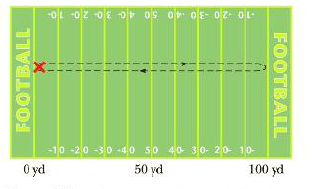Chapter 2.1, Problem 2.1QQ

Chapter
Section
Textbook Problem

Figure 2.4 shows the unusual path of a confused football player. After receiving a kickoff at his own goal, he runs downfield to within inches of a touchdown, then reverses direction and races back until he’s tackled at the exact location where he first caught the ball. During this run, which took 25 s, what is (a) the path length he travels, (b) his displacement, (c) his average velocity in the x-direction, and (d) his average speed?Figure 2.4 (Quick Quiz 2.1) The path followed by a confused football player.

(a)

To determine
The total path length of the football player.

Explanation

Given Info: The length of the ground is 100yd .

Explanation:

The football player moves forward from one end of the ground and comes back to the starting point after reaching the other end. The total path length covered will be twice the length of the ground.

The total distance covered by the football player is,

d=2x

Here,

d is the path length.

x is the length of the ground

(b)

To determine
The displacement of the football player.

(c)

To determine
The average velocity of the football player.

(d)

To determine
The average speed of the football player.

Still sussing out bartleby?

Check out a sample textbook solution.

See a sample solution

The Solution to Your Study Problems

Bartleby provides explanations to thousands of textbook problems written by our experts, many with advanced degrees!

Get Started